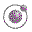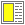Name binding, Recursion, Iteration, and Continuations - slide 3 : 42

The equivalent meaning of let
We will here understand the underlying, equivalent form of a let name binding construct
```(let ((n1 e1)
...
(nk ek))
body-expr)```
```((lambda (n1 ... nk) body-expr)
e1 ... ek)```